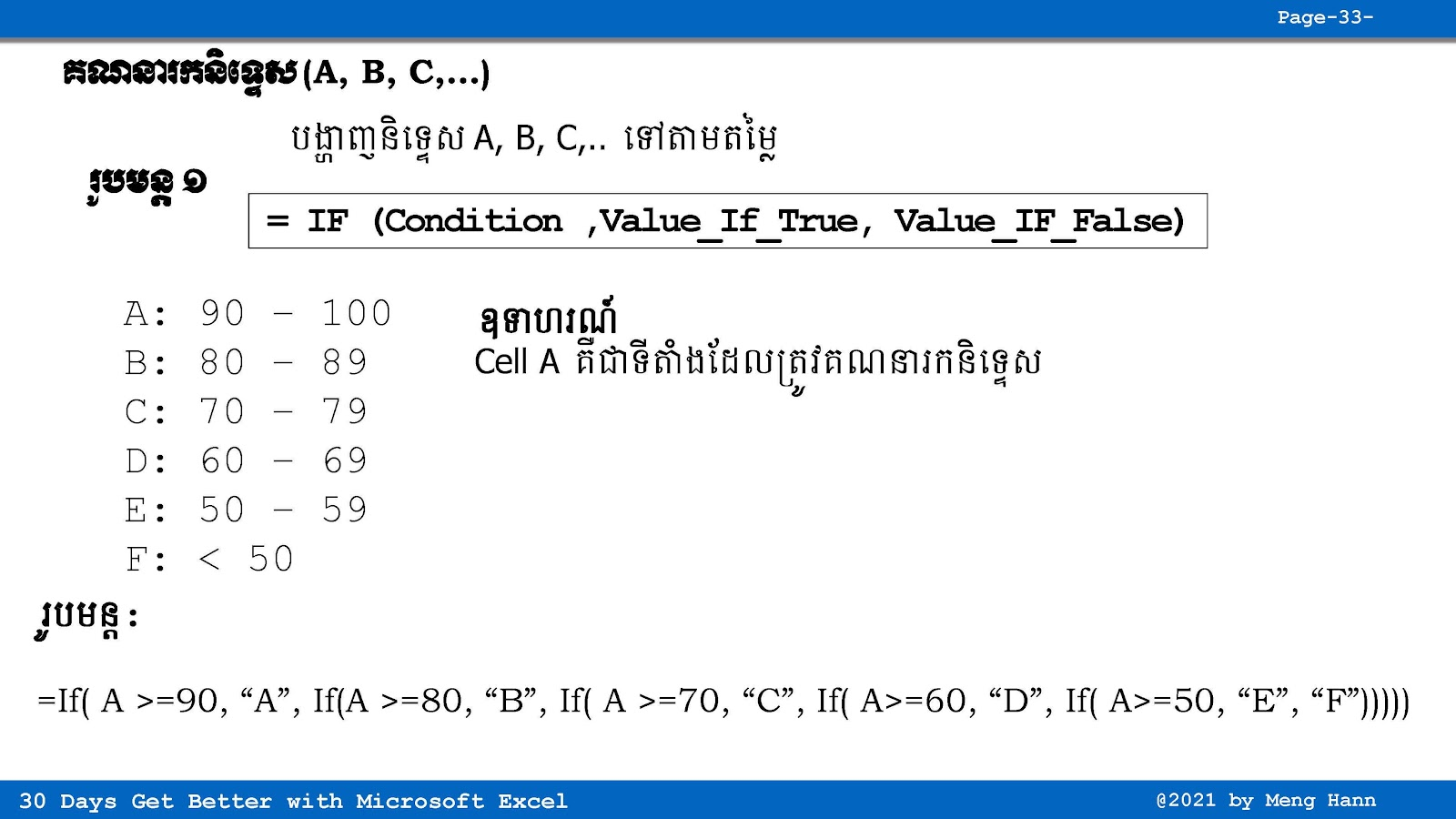# 28. How to Calculate Grade (A, B, C,..) in Excel speak Khmer

28. Calculate Grade (A, B, C,..) in Excel speak Khmer
(រូបមន្ត IF ក្នុង IF គណនាចំណាត់ថ្នាក់ A, B, C,...) If you want to calculate grade in Excel, you can use IF formula to do that but it is a long sentence. This is nested IF (IF in IF).